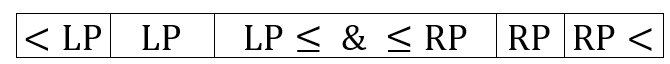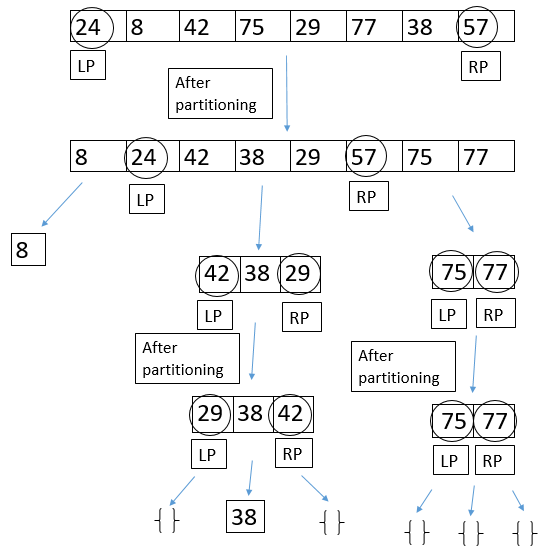Related Articles

# Dual pivot Quicksort

• Difficulty Level : Medium
• Last Updated : 11 Jun, 2021

As we know, the single pivot quick sort takes a pivot from one of the ends of the array and partitioning the array, so that all elements are left to the pivot are less than or equal to the pivot, and all elements that are right to the pivot are greater than the pivot.
The idea of dual pivot quick sort is to take two pivots, one in the left end of the array and the second, in the right end of the array. The left pivot must be less than or equal to the right pivot, so we swap them if necessary.
Then, we begin partitioning the array into three parts: in the first part, all elements will be less than the left pivot, in the second part all elements will be greater or equal to the left pivot and also will be less than or equal to the right pivot, and in the third part all elements will be greater than the right pivot. Then, we shift the two pivots to their appropriate positions as we see in the below bar, and after that we begin quicksorting these three parts recursively, using this method.Dual pivot quick sort is a little bit faster than the original single pivot quicksort. But still, the worst case will remain O(n^2) when the array is already sorted in an increasing or decreasing order.
An example:## C++

 `// C++ program to implement dual pivot QuickSort ` `#include ` `using` `namespace` `std; ` ` `  `int` `partition(``int``* arr, ``int` `low, ``int` `high, ``int``* lp); ` ` `  `void` `swap(``int``* a, ``int``* b) ` `{ ` `    ``int` `temp = *a; ` `    ``*a = *b; ` `    ``*b = temp; ` `} ` ` `  `void` `DualPivotQuickSort(``int``* arr, ``int` `low, ``int` `high) ` `{ ` `    ``if` `(low < high) { ` `        ``// lp means left pivot, and rp means right pivot. ` `        ``int` `lp, rp; ` `        ``rp = partition(arr, low, high, &lp); ` `        ``DualPivotQuickSort(arr, low, lp - 1); ` `        ``DualPivotQuickSort(arr, lp + 1, rp - 1); ` `        ``DualPivotQuickSort(arr, rp + 1, high); ` `    ``} ` `} ` ` `  `int` `partition(``int``* arr, ``int` `low, ``int` `high, ``int``* lp) ` `{ ` `    ``if` `(arr[low] > arr[high]) ` `        ``swap(&arr[low], &arr[high]); ` ` `  `    ``// p is the left pivot, and q is the right pivot. ` `    ``int` `j = low + 1; ` `    ``int` `g = high - 1, k = low + 1, p = arr[low], q = arr[high]; ` `    ``while` `(k <= g) { ` ` `  `        ``// if elements are less than the left pivot ` `        ``if` `(arr[k] < p) { ` `            ``swap(&arr[k], &arr[j]); ` `            ``j++; ` `        ``} ` ` `  `        ``// if elements are greater than or equal ` `        ``// to the right pivot ` `        ``else` `if` `(arr[k] >= q) { ` `            ``while` `(arr[g] > q && k < g) ` `                ``g--; ` `            ``swap(&arr[k], &arr[g]); ` `            ``g--; ` `            ``if` `(arr[k] < p) { ` `                ``swap(&arr[k], &arr[j]); ` `                ``j++; ` `            ``} ` `        ``} ` `        ``k++; ` `    ``} ` `    ``j--; ` `    ``g++; ` ` `  `    ``// bring pivots to their appropriate positions. ` `    ``swap(&arr[low], &arr[j]); ` `    ``swap(&arr[high], &arr[g]); ` ` `  `    ``// returning the indices of the pivots. ` `    ``*lp = j; ``// because we cannot return two elements ` `    ``// from a function. ` ` `  `    ``return` `g; ` `} ` ` `  `// Driver code ` `int` `main() ` `{ ` `    ``int` `arr[] = { 24, 8, 42, 75, 29, 77, 38, 57 }; ` `    ``DualPivotQuickSort(arr, 0, 7); ` `    ``cout << ``"Sorted array: "``; ` `    ``for` `(``int` `i = 0; i < 8; i++) ` `        ``cout << arr[i] << ``" "``; ` `    ``cout << endl; ` `} ` ` `  `// This code is contributed by SHUBHAMSINGH10 `

## C

 `// C program to implement dual pivot QuickSort ` `#include ` ` `  `int` `partition(``int``* arr, ``int` `low, ``int` `high, ``int``* lp); ` ` `  `void` `swap(``int``* a, ``int``* b) ` `{ ` `    ``int` `temp = *a; ` `    ``*a = *b; ` `    ``*b = temp; ` `} ` ` `  `void` `DualPivotQuickSort(``int``* arr, ``int` `low, ``int` `high) ` `{ ` `    ``if` `(low < high) { ` `        ``// lp means left pivot, and rp means right pivot. ` `        ``int` `lp, rp; ` `        ``rp = partition(arr, low, high, &lp); ` `        ``DualPivotQuickSort(arr, low, lp - 1); ` `        ``DualPivotQuickSort(arr, lp + 1, rp - 1); ` `        ``DualPivotQuickSort(arr, rp + 1, high); ` `    ``} ` `} ` ` `  `int` `partition(``int``* arr, ``int` `low, ``int` `high, ``int``* lp) ` `{ ` `    ``if` `(arr[low] > arr[high]) ` `        ``swap(&arr[low], &arr[high]); ` `    ``// p is the left pivot, and q is the right pivot. ` `    ``int` `j = low + 1; ` `    ``int` `g = high - 1, k = low + 1, p = arr[low], q = arr[high]; ` `    ``while` `(k <= g) { ` ` `  `        ``// if elements are less than the left pivot ` `        ``if` `(arr[k] < p) { ` `            ``swap(&arr[k], &arr[j]); ` `            ``j++; ` `        ``} ` ` `  `        ``// if elements are greater than or equal ` `        ``// to the right pivot ` `        ``else` `if` `(arr[k] >= q) { ` `            ``while` `(arr[g] > q && k < g) ` `                ``g--; ` `            ``swap(&arr[k], &arr[g]); ` `            ``g--; ` `            ``if` `(arr[k] < p) { ` `                ``swap(&arr[k], &arr[j]); ` `                ``j++; ` `            ``} ` `        ``} ` `        ``k++; ` `    ``} ` `    ``j--; ` `    ``g++; ` ` `  `    ``// bring pivots to their appropriate positions. ` `    ``swap(&arr[low], &arr[j]); ` `    ``swap(&arr[high], &arr[g]); ` ` `  `    ``// returning the indices of the pivots. ` `    ``*lp = j; ``// because we cannot return two elements ` `    ``// from a function. ` ` `  `    ``return` `g; ` `} ` ` `  `// Driver code ` `int` `main() ` `{ ` `    ``int` `arr[] = { 24, 8, 42, 75, 29, 77, 38, 57 }; ` `    ``DualPivotQuickSort(arr, 0, 7); ` `    ``printf``(``"Sorted array: "``); ` `    ``for` `(``int` `i = 0; i < 8; i++) ` `        ``printf``(``"%d "``, arr[i]); ` `    ``printf``(``"\n"``); ` `    ``return` `0; ` `} `

## Java

 `// Java program to implement ` `// dual pivot QuickSort ` `class` `GFG{ ` ` `  `static` `void` `swap(``int``[] arr, ``int` `i, ``int` `j) ` `{ ` `    ``int` `temp = arr[i]; ` `    ``arr[i] = arr[j]; ` `    ``arr[j] = temp; ` `} ` ` `  `static` `void` `dualPivotQuickSort(``int``[] arr,  ` `                               ``int` `low, ``int` `high) ` `{ ` `    ``if` `(low < high) ` `    ``{ ` `         `  `        ``// piv[] stores left pivot and right pivot. ` `        ``// piv means left pivot and ` `        ``// piv means right pivot ` `        ``int``[] piv; ` `        ``piv = partition(arr, low, high); ` `         `  `        ``dualPivotQuickSort(arr, low, piv[``0``] - ``1``); ` `        ``dualPivotQuickSort(arr, piv[``0``] + ``1``, piv[``1``] - ``1``); ` `        ``dualPivotQuickSort(arr, piv[``1``] + ``1``, high); ` `    ``} ` `} ` ` `  `static` `int``[] partition(``int``[] arr, ``int` `low, ``int` `high) ` `{ ` `    ``if` `(arr[low] > arr[high]) ` `        ``swap(arr, low, high); ` `         `  `    ``// p is the left pivot, and q  ` `    ``// is the right pivot. ` `    ``int` `j = low + ``1``; ` `    ``int` `g = high - ``1``, k = low + ``1``, ` `        ``p = arr[low], q = arr[high]; ` `         `  `    ``while` `(k <= g)  ` `    ``{ ` `         `  `        ``// If elements are less than the left pivot ` `        ``if` `(arr[k] < p) ` `        ``{ ` `            ``swap(arr, k, j); ` `            ``j++; ` `        ``} ` `         `  `        ``// If elements are greater than or equal ` `        ``// to the right pivot ` `        ``else` `if` `(arr[k] >= q)  ` `        ``{ ` `            ``while` `(arr[g] > q && k < g) ` `                ``g--; ` `                 `  `            ``swap(arr, k, g); ` `            ``g--; ` `             `  `            ``if` `(arr[k] < p) ` `            ``{ ` `                ``swap(arr, k, j); ` `                ``j++; ` `            ``} ` `        ``} ` `        ``k++; ` `    ``} ` `    ``j--; ` `    ``g++; ` `     `  `    ``// Bring pivots to their appropriate positions. ` `    ``swap(arr, low, j); ` `    ``swap(arr, high, g); ` ` `  `    ``// Returning the indices of the pivots ` `    ``// because we cannot return two elements ` `    ``// from a function, we do that using an array. ` `    ``return` `new` `int``[] { j, g }; ` `} ` ` `  `// Driver code ` `public` `static` `void` `main(String[] args) ` `{ ` `    ``int``[] arr = { ``24``, ``8``, ``42``, ``75``, ``29``, ``77``, ``38``, ``57` `}; ` `     `  `    ``dualPivotQuickSort(arr, ``0``, ``7``); ` `     `  `    ``System.out.print(``"Sorted array: "``); ` `    ``for` `(``int` `i = ``0``; i < ``8``; i++) ` `        ``System.out.print(arr[i] + ``" "``); ` `         `  `    ``System.out.println(); ` `} ` `} ` ` `  `// This code is contributed by Gourish Sadhu `

## Python3

 `# Python3 program to implement  ` `# dual pivot QuickSort ` `def` `dualPivotQuickSort(arr, low, high): ` `     `  `    ``if` `low < high: ` `         `  `        ``# lp means left pivot and rp  ` `        ``# means right pivot ` `        ``lp, rp ``=` `partition(arr, low, high) ` `         `  `        ``dualPivotQuickSort(arr, low, lp ``-` `1``) ` `        ``dualPivotQuickSort(arr, lp ``+` `1``, rp ``-` `1``) ` `        ``dualPivotQuickSort(arr, rp ``+` `1``, high) ` `         `  `def` `partition(arr, low, high): ` `     `  `    ``if` `arr[low] > arr[high]: ` `        ``arr[low], arr[high] ``=` `arr[high], arr[low] ` `         `  `    ``# p is the left pivot, and q is the right pivot. ` `    ``j ``=` `k ``=` `low ``+` `1` `    ``g, p, q ``=` `high ``-` `1``, arr[low], arr[high] ` `     `  `    ``while` `k <``=` `g: ` `         `  `        ``# If elements are less than the left pivot ` `        ``if` `arr[k] < p: ` `            ``arr[k], arr[j] ``=` `arr[j], arr[k] ` `            ``j ``+``=` `1` `             `  `        ``# If elements are greater than or equal  ` `        ``# to the right pivot ` `        ``elif` `arr[k] >``=` `q: ` `            ``while` `arr[g] > q ``and` `k < g: ` `                ``g ``-``=` `1` `                 `  `            ``arr[k], arr[g] ``=` `arr[g], arr[k] ` `            ``g ``-``=` `1` `             `  `            ``if` `arr[k] < p: ` `                ``arr[k], arr[j] ``=` `arr[j], arr[k] ` `                ``j ``+``=` `1` `                 `  `        ``k ``+``=` `1` `         `  `    ``j ``-``=` `1` `    ``g ``+``=` `1` `     `  `    ``# Bring pivots to their appropriate positions. ` `    ``arr[low], arr[j] ``=` `arr[j], arr[low] ` `    ``arr[high], arr[g] ``=` `arr[g], arr[high] ` `     `  `    ``# Returning the indices of the pivots ` `    ``return` `j, g ` ` `  `# Driver code ` `arr ``=` `[ ``24``, ``8``, ``42``, ``75``, ``29``, ``77``, ``38``, ``57` `] ` `dualPivotQuickSort(arr, ``0``, ``7``) ` ` `  `print``(``'Sorted array: '``, end ``=` `'') ` `for` `i ``in` `arr: ` `    ``print``(i, end ``=` `' '``) ` `     `  `print``() ` `     `  `# This code is contributed by Gourish Sadhu `

Output:

```Sorted array: 8 24 29 38 42 57 75 77
```

This article is contributed by Shlomi Elhaiani. If you like GeeksforGeeks and would like to contribute, you can also write an article using write.geeksforgeeks.org or mail your article to review-team@geeksforgeeks.org. See your article appearing on the GeeksforGeeks main page and help other Geeks.